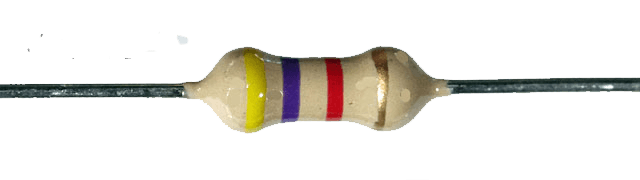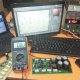Breaking News
Home / Electronic component / What is the resistor? how it’s work?

# What is the resistor? how it’s work?

## What is the resistor? how it’s work:

The resistor is the simplest components in any circuit or electronics. Resistors come in many different sizes and shapes. Their work is to restrict the flow of electrons and reduce the current or voltage flowing by converting electrical energy into heat. In the audio system, volume controls in audio equipment use variable resistors like these. volume is also a resistor. Every resistor has its value number. every electronics component has there value number. normally we can find every component value number to there body. But the only resistor has a different system to find out their value number. it uses the color code to find out the value number. What is the resistor? how it’s work?

First, we have to know the color serial number

### The Resistor Colour Code

 Colour Digit Multiplier Tolerance Black 0 1 Brown 1 10 ± 1% Red 2 100 ± 2% Orange 3 1,000 Yellow 4 10,000 Green 5 100,000 ± 0.5% Blue 6 1,000,000 ± 0.25% Violet 7 10,000,000 ± 0.1% Grey 8 ± 0.05% White 9 Gold 0.1 ± 5% Silver 0.01 ± 10% None ± 20%

For an example,### Digit, Digit, Multiplier = Colour, Colour X multipler colour  in Ohm’s (Ω)

yellow Violet Red = 4 7 2 = 4 7 x 102 = 4700Ω or 4k7 Ohm and Gold is for 5%. normally we don’t count the Gold value.

Now we can understand that always Gold will be the last number when we will calculate the number we must count the first 2 color number 3rd color number we don’t count. for the 3rd number will be multipler number. we can see the example, first 2 colors we take yellow=4, and violet=7 and for red? for red we take multipler n

### BS 1852 Codes for Resistor Values

0.47Ω = R47 or 0R47
1.0Ω = 1R0
4.7Ω = 4R7
47Ω = 47R
470Ω = 470R or 0K47
1.0KΩ = 1K0
4.7KΩ = 4K7
47KΩ = 47K
470KΩ = 470K or 0M47
1MΩ = 1M0

## Value X Multiplier = Resistance

3.3 x 1 = 3.3Ω

3.3 x 10 = 33Ω

3.3 x 100 = 330Ω

3.3 x 1,000 = 3.3kΩ

3.3 x 10,000 = 33kΩ

3.3 x 100,000 = 330kΩ

3.3 x 1,000,000 = 3.3MΩ

We try to explen the resistor color code. it’s vary easy system to learn the resistor color code. first we need to know the color code number. here in 4 color we had to use first 2 color number 3rd color number no need. 3rd number need the multiplier number. Gold is for 5% + or 5% -.

transistor circuit diagram of 2sa1943 and 2sc5200

Thanks a lot to be with us. If you want another post then please visit our website.

We have another post for you. like as repairing amplifier,